A fast library for increasing the number of training images by applying various transformations.

# Augmentor.jl’s documentation¶

Augmentor is a real-time image augmentation library designed to render the process of artificial dataset enlargement more convenient, less error prone, and easier to reproduce. It offers the user the ability to build a stochastic augmentation pipeline using simple building blocks. In other words, a stochastic augmentation pipeline is simply a sequence of operations for which the parameters can (but need not) be random variables as the following code snippet demonstrates.

julia> pipeline = Rotate([-5, -3, 0, 3, 5]) |> CropSize(64, 64) |> Zoom(1:0.1:1.2)
3-step Augmentor.ImmutablePipeline:
1.) Rotate by θ ∈ [-5, -3, 0, 3, 5] degree
2.) Crop a 64×64 window around the center
3.) Zoom by I ∈ {1.0×1.0, 1.1×1.1, 1.2×1.2}


The Julia version of Augmentor is engineered specifically for high performance applications. It makes use of multiple heuristics to generate efficient tailor-made code for the concrete user-specified augmentation pipeline. In particular Augmentor tries to avoid the need for any intermediate images, but instead aims to compute the output image directly from the input in one single pass.

## Where to begin?¶

If this is the first time you consider using Augmentor.jl for your machine learning related experiments or packages, make sure to check out the “Getting Started” section. There we list the installation instructions and some simple hello world examples.

### Getting Started¶

In this section we will provide a condensed overview of the package. In order to keep this overview concise, we will not discuss any background information or theory on the losses here in detail.

#### Installation¶

To install Augmentor.jl, start up Julia and type the following code-snipped into the REPL. It makes use of the native Julia package manger.

Pkg.add("Augmentor")


Additionally, for example if you encounter any sudden issues, or in the case you would like to contribute to the package, you can manually choose to be on the latest (untagged) version.

Pkg.checkout("Augmentor")


#### Getting Help¶

To get help on specific functionality you can either look up the information here, or if you prefer you can make use of Julia’s native doc-system. The following example shows how to get additional information on augment within Julia’s REPL:

?augment


Augmentor.jl is the Julia package for Augmentor. You can find the Python version here .

## Introduction and Motivation¶

If you are new to image augmentation in general, or are simply interested in some background information, feel free to take a look at the following sections. There we discuss the concepts involved and outline the most important terms and definitions.

### Background and Motivation¶

In this section we will discuss the concept of image augmentation in general. In particular we will introduce some terminology and useful definitions.

#### What is Image Augmentation?¶

The term data augmentation is commonly used to describe the process of repeatedly applying various transformations to some dataset, with the hope that the output (i.e. the newly generated observations) bias the model towards learning better features. Depending on the structure and semantics of the data, coming up with such transformations can be a challenge by itself.

Images are a special class of data that exhibit some interesting properties in respect to their structure. For example do the dimensions of an image (i.e. the pixel) exhibit a spatial relationship to each other. As such, a lot of commonly used augmentation strategies for image data revolve around affine transformations, such as translations or rotations. Because images are such a popular and special case of data, they deserve their own sub-category of data augmentation, which we will unsurprisingly refer to as image augmentation.

The general idea is the following: if we want our model to generalize well, then we should design the learning process in such a way as to bias the model into learning such transformation-equivariant properties. One way to do this is via the design of the model itself, which for example was idea behind convolutional neural networks. An orthogonal approach to bias the model to learn about this equivariance - and the focus of this package - is by using label-preserving transformations.

#### Label-preserving Transformations¶

Before attempting to train a model using some augmentation pipeline, it’s a good idea to invest some time in deciding on an appropriate set of transformations to choose from. Some of these transformations also have parameters to tune, and we should also make sure that we settle on a decent set of values for those.

What constitutes as “decent” depends on the dataset. In general we want the augmented images to be fairly dissimilar to the originals. However, we need to be careful that the augmented images still visually represent the same concept (and thus label). If a pipeline only produces output images that have this property we call this pipeline label-preserving.

##### Example: MNIST Handwritten Digits¶

Consider the following example from the MNIST database of handwritten digits [MNIST1998]. Our input image clearly represents its associated label “6”. If we were to use the transformation Rotate180 in our augmentation pipeline for this type of images, we could end up with the situation depicted by the image on the right side.

using Augmentor, Images, MNIST
input_img  = MNIST.trainimage(19)
output_img = augment(input_img, Rotate180())

Input Output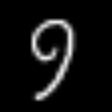To a human, this newly transformed image clearly represents the label “9”, and not “6” like the original image did. In image augmentation, however, the assumption is that the output of the pipeline has the same label as the input. That means that in this example we would tell our model that the correct answer for the image on the right side is “6”, which is clearly undesirable for obvious reasons.

Thus, for the MNIST dataset, the transformation Rotate180 is not label-preserving and should not be used for augmentation.

 [MNIST1998] LeCun, Yan, Corinna Cortes, Christopher J.C. Burges. “The MNIST database of handwritten digits” Website. 1998.
##### Example: ISIC Skin Lesions¶

On the other hand, the exact same transformation could very well be label-preserving for other types of images. Let us take a look at a different set of image data; this time from the medical domain.

The International Skin Imaging Collaboration [ISIC] hosts a large collection of publicly available and labeled skin lesion images. A subset of that data was used in 2016’s ISBI challenge [ISBI2016] where a subtask was lesion classification.

Let’s consider the following input image on the left side. It shows a photo of a skin lesion that was taken from above. By applying the Rotate180 operation to the input image, we end up with a transformed version shown on the right side.

using Augmentor, ISICArchive
input_img  = get(ImageThumbnailRequest(id = "5592ac599fc3c13155a57a85"))
output_img = augment(input_img, Rotate180())

Input Output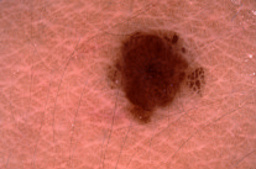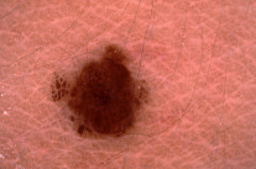After looking at both images, one could argue that the orientation of the camera is somewhat arbitrary as long as it points to the lesion at an approximately orthogonal angle. Thus, for the ISIC dataset, the transformation Rotate180 could be considered as label-preserving and very well be tried for augmentation. Of course this does not guarantee that it will improve training time or model accuracy, but the point is that it is unlikely to hurt.

 [ISBI2016] Gutman, David; Codella, Noel C. F.; Celebi, Emre; Helba, Brian; Marchetti, Michael; Mishra, Nabin; Halpern, Allan. “Skin Lesion Analysis toward Melanoma Detection: A Challenge at the International Symposium on Biomedical Imaging (ISBI) 2016, hosted by the International Skin Imaging Collaboration (ISIC)”. eprint arXiv:1605.01397. 2016.

In case you have not worked with image data in Julia before, feel free to browse the following documents for a crash course on how image data is represented in the Julia language, as well as how to visualize it.

### Working with Images in Julia¶

The Julia language provides a rich syntax as well as large set of highly-optimized functionality for working with (multi-dimensional) arrays of what is known as “bit types” or compositions of such. Because of this, the language lends itself particularly well to the fairly simple idea of treating images as just plain arrays. Even though this may sound as a rather tedious low-level approach, Julia makes it possible to still allow for powerful abstraction layers without the loss of generality that usually comes with that. This is accomplished with help of Julia’s flexible type system and multiple dispatch (both of which are beyond the scope of this tutorial).

While the images-are-arrays-approach makes working with images in Julia very performant, it has also been source of confusion to new community members. This beginner’s guide is an attempt to provide a step-by-step overview of how pixel data is handled in Julia. To get a more detailed explanation on some particular concept involved, please take a look at the documentation of the JuliaImages ecosystem.

#### Multi-dimensional Arrays¶

To wrap our heads around Julia’s array-based treatment of images, we first need to understand what Julia arrays are and how we can work with them.

Note

This section is only intended provide a simplified and thus partial overview of Julia’s arrays capabilities in order to gain some intuition about pixel data. For a more detailed treatment of the topic please have a look at the official documentation

Whenever we work with an Array in which the elements are bit-types (e.g. Int64, Float32, UInt8, etc), we can think of the array as a continuous block of memory. This is useful for many different reasons, such as cache locality and interacting with external libraries.

The same block of memory can be interpreted in a number of ways. Consider the following example in which we allocate a vector (i.e. a one dimensional array) of UInt8 (i.e. bytes) with some ordered example values ranging from 1 to 6. We will think of this as our physical memory block, since it is a pretty close representation.

julia> memory = [0x1, 0x2, 0x3, 0x4, 0x5, 0x6]
6-element Array{UInt8,1}:
0x01
0x02
0x03
0x04
0x05
0x06


The same block of memory could also be interpreted differently. For example we could think of this as a matrix with 3 rows and 2 columns instead (or even the other way around). The function reinterpret allows us to do just that

julia> A = reinterpret(UInt8, memory, (3,2))
3x2 Array{UInt8,2}:
0x01  0x04
0x02  0x05
0x03  0x06


Note how we specified the number of rows first. This is because the Julia language follows the column-major convention for multi dimensional arrays. What this means can be observed when we compare our new matrix A with the initial vector memory and look at the element layout. Both variables are using the same underlying memory (i.e the value 0x01 is physically stored right next to the value 0x02 in our example, while 0x01 and 0x04 are quite far apart even though the matrix interpretation makes it look like they are neighbors; which they are not).

Tip

A quick and dirty way to check if two variables are representing the same block of memory is by comparing the output of pointer(myvariable). Note, however, that technically this only tells you where a variable starts in memory and thus has its limitations.

This idea can also be generalized for higher dimensions. For example we can think of this as a 3D array as well.

julia> reinterpret(UInt8, memory, (3,1,2))
3x1x2 Array{UInt8,3}:
[:, :, 1] =
0x01
0x02
0x03

[:, :, 2] =
0x04
0x05
0x06


If you take a closer look at the dimension sizes, you can see that all we did in that example was add a new dimension of size 1, while not changing the other numbers. In fact we can add any number of practically empty dimensions, otherwise known as singleton dimensions.

reinterpret(UInt8, memory, (3,1,1,1,2))
3x1x1x1x2 Array{UInt8,5}:
[:, :, 1, 1, 1] =
0x01
0x02
0x03

[:, :, 1, 1, 2] =
0x04
0x05
0x06


This is a useful property to have when we are confronted with greyscale datasets that do not have a color channel, yet we still want to work with a library that expects the images to have one.

#### Vertical-Major vs Horizontal-Major¶

There are a number of different conventions for how to store image data into a binary format. The first question one has to address is the order in which the image dimensions are transcribed.

We have seen before that Julia follows the column-major convention for its arrays, which for images would lead to the corresponding convention of being vertical-major. In the image domain, however, it is fairly common to store the pixels in a horizontal-major layout. In other words, horizontal-major means that images are stored in memory (or file) one pixel row after the other.

In most cases, when working within the JuliaImages ecosystem, the images should already be in the Julia-native column major layout. If for some reason that is not the case there are two possible ways to convert the image to that format.

julia> At = reinterpret(UInt8, memory, (3,2))' # "row-major" layout
2×3 Array{UInt8,2}:
0x01  0x02  0x03
0x04  0x05  0x06

1. The first way to alter the pixel order is by using the function Base.permutedims. In contrast to what we have seen before, this function will allocate a new array and copy the values in the appropriate manner.

julia> B = permutedims(At, (2, 1))
3×2 Array{UInt8,2}:
0x01  0x04
0x02  0x05
0x03  0x06

2. The second way is using the function ImageCore.permuteddimsview which results in a lazy view that does not allocate a new array but instead only computes the correct values when queried.

julia> using ImageCore

julia> C = permuteddimsview(At, (2, 1))
3×2 permuteddimsview(::Array{UInt8,2}, (2,1)) with element type UInt8:
0x01  0x04
0x02  0x05
0x03  0x06


Either way, it is in general a good idea to make sure that the array one is working with ends up in a column-major layout.

#### Reinterpreting Elements¶

Up to this point, all we talked about was how to reinterpreting or permuting the dimensional layout of some continuous memory block. If you look at the examples above you will see that all the arrays have elements of type UInt8, which just means that each element is represented by a single byte in memory.

Knowing all this, we can now take the idea a step further and think about reinterpreting the element types of the array. Let us consider our original vector memory again.

julia> memory = [0x1, 0x2, 0x3, 0x4, 0x5, 0x6]
6-element Array{UInt8,1}:
0x01
0x02
0x03
0x04
0x05
0x06


Note how each byte is thought of as an individual element. One thing we could do instead, is think of this memory block as a vector of 3 UInt16 elements.

julia> reinterpret(UInt16, memory)
3-element Array{UInt16,1}:
0x0201
0x0403
0x0605


Pay attention to where our original bytes ended up. In contrast to just rearranging elements as we did before, we ended up with significantly different element values. One may ask why it would ever be practical to reinterpret a memory block like this. The one word answer to this is Colors! As we will see in the remainder of this tutorial, it turns out to be a very useful thing to do when your arrays represent pixel data.

#### Introduction to Color Models¶

As we discussed before, there are a various number of conventions on how to store pixel data into a binary format. That is not only true for dimension priority, but also for color information.

One way color information can differ is in the color model in which they are described in. Two famous examples for color models are RGB and HSV. They essentially define how colors are conceptually made up in terms of some components. Additionally, one can decide on how many bits to use to describe each color component. By doing so one defines the available color depth.

Before we look into using the actual implementation of Julia’s color models, let us prototype our own imperfect toy model in order to get a better understanding of what is happening under the hood.

# define our toy color model
immutable MyRGB
r::UInt8
b::UInt8
g::UInt8
end


Note how we defined our new toy color model as immutable. Because of this and the fact that all its components are bit types (in this case UInt8), any instantiation of our new type will be represented as a continuous block of memory as well.

We can now apply our color model to our memory vector from above, and interpret the underlying memory as a vector of to MyRGB values instead.

julia> reinterpret(MyRGB, memory)
2-element Array{MyRGB,1}:
MyRGB(0x01,0x02,0x03)
MyRGB(0x04,0x05,0x06)


Similar to the UInt16 example, we now group neighboring bytes into larger units (namely MyRGB). In contrast to the UInt16 example we are still able to access the individual components underneath. This simple toy color model already allows us to do a lot of useful things. We could define functions that work on MyRGB values in a color-space appropriate fashion. We could also define other color models and implement function to convert between them.

However, our little toy color model is not yet optimal. For example it hard-codes a predefined color depth of 24 bit. We may have use-cases where we need a richer color space. One thing we could do to achieve that would be to introduce a new type in similar fashion. Still, because they have a different range of available numbers per channel (because they have a different amount of bits per channel), we would have to write a lot of specialized code to be able to appropriately handle all color models and depth.

Luckily, the creators of ColorTypes.jl went a with a more generic strategy: Using parameterized types and fixed point numbers.

Tip

If you are interested in how various color models are actually designed and/or implemented in Julia, you can take a look at the ColorTypes.jl package

#### Fixed Point Numbers¶

The idea behind using fixed point numbers for each color component is fairly simple. No matter how many bits a component is made up of, we always want the largest possible value of the component to be equal to 1.0 and the smallest possible value to be equal to 0. Of course, the amount of possible intermediate numbers still depends on the number of underlying bits in the memory, but that is not much of an issue.

julia> reinterpret(UFixed8, 0xFF)
UFixed8(1.0)

julia> reinterpret(UFixed16, 0xFFFF)
UFixed16(1.0)


Not only does this allow for simple conversion between different color depths, it also allows us to implement generic algorithms, that are completely agnostic to the utilized color depth.

It is worth pointing out again, that we get all these goodies without actually changing or copying the original memory block. Remember how during this whole tutorial we have only changed the interpretation of some underlying memory, and have not had the need to copy any data so far.

Tip

For pixel data we are mainly interested in unsigned fixed point numbers, but there are others too. Check out the package FixedPointNumbers.jl for more information on fixed point numbers in general.

Let us now leave our toy model behind and use the actual implementation of RGB on our example vector memory. With the first command we will interpret our data as two pixels with 8 bit per color channel, and with the second command as a single pixel of 16 bit per color channel

julia> reinterpret(RGB{UFixed8}, memory)
2-element Array{ColorTypes.RGB{FixedPointNumbers.UFixed{UInt8,8}},1}:
RGB{UFixed8}(0.004,0.008,0.012)
RGB{UFixed8}(0.016,0.02,0.024)

julia> reinterpret(RGB{UFixed16}, memory)
1-element Array{ColorTypes.RGB{FixedPointNumbers.UFixed{UInt16,16}},1}:
RGB{UFixed16}(0.00783,0.01567,0.02351)


Note how the values are now interpreted as floating point numbers.

## User’s Guide¶

Augmentor provides a number of already implemented functionality. The following section provides a complete list of all the exported operations and their documentation.

### Supported Operations¶

This page lists and describes all supported image operations, and is mainly intended as a quick preview of the available functionality.

Category Available Operations
Mirroring FlipX FlipY
Rotating Rotate90 Rotate270 Rotate180 Rotate
Shearing ShearX ShearY
Distorting ElasticDistortion
Scaling and Resizing Scale Zoom Resize
Cropping Crop CropNative CropSize CropRatio RCropRatio
Conversion ConvertEltype
Information Layout SplitChannels CombineChannels PermuteDims Reshape
Utility Operations NoOp CacheImage Either

#### Affine Transformations¶

A good portion of the provided operations fall under the category of affine transformations. As such, they can be described using what is known as an affine map, which are inherently compose-able if chained together. However, utilizing such a affine formulation requires (costly) interpolation, which may not always be needed to achieve the desired effect. For that reason do some of the operations below also provide a special purpose implementation to produce their specified result. Those are usually preferred over the affine formulation if sensible considering the complete pipeline.

##### Mirroring¶
class FlipX

Reverses the x-order of each pixel row. Another way of describing it would be to mirror the image on the y-axis, or to mirror the image horizontally.

julia> FlipX()
Flip the X axis

julia> FlipX(0.3)
Augmentor.Either (1 out of 2 operation(s)):
- 30% chance to: Flip the X axis
- 70% chance to: No operation

Input Output for FlipX()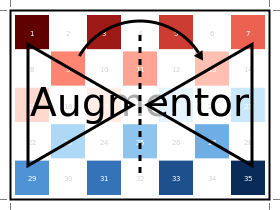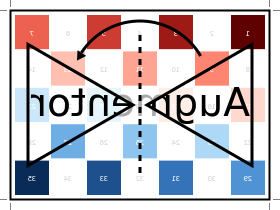class FlipY

Reverses the y-order of each pixel column. Another way of describing it would be to mirror the image on the x-axis, or to mirror the image vertically.

julia> FlipY()
Flip the Y axis

julia> FlipY(0.3)
Augmentor.Either (1 out of 2 operation(s)):
- 30% chance to: Flip the Y axis
- 70% chance to: No operation

Input Output for FlipY()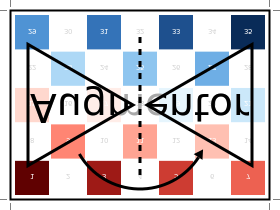##### Rotating¶
class Rotate90

Rotates the image upwards 90 degrees. This is a special case rotation because it can be performed very efficiently by simply rearranging the existing pixels. However, it is generally not the case that the output image will have the same size as the input image, which is something to be aware of.

julia> Rotate90()
Rotate 90 degree

julia> Rotate90(0.3)
Augmentor.Either (1 out of 2 operation(s)):
- 30% chance to: Rotate 90 degree
- 70% chance to: No operation

Input Output for Rotate90()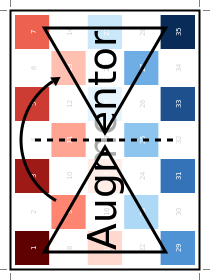class Rotate180

Rotates the image 180 degrees. This is a special case rotation because it can be performed very efficiently by simply rearranging the existing pixels. Furthermore, the output image will have the same dimensions as the input image.

julia> Rotate180()
Rotate 180 degree

julia> Rotate180(0.3)
Augmentor.Either (1 out of 2 operation(s)):
- 30% chance to: Rotate 180 degree
- 70% chance to: No operation

Input Output for Rotate180()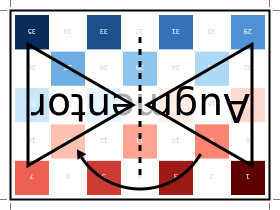class Rotate270

Rotates the image upwards 270 degrees, which can also be described as rotating the image downwards 90 degrees. This is a special case rotation, because it can be performed very efficiently by simply rearranging the existing pixels. However, it is generally not the case that the output image will have the same size as the input image, which is something to be aware of.

julia> Rotate270()
Rotate 270 degree

julia> Rotate270(0.3)
Augmentor.Either (1 out of 2 operation(s)):
- 30% chance to: Rotate 270 degree
- 70% chance to: No operation

Input Output for Rotate270()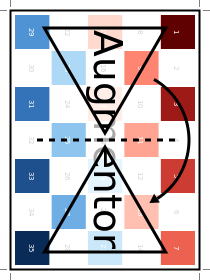class Rotate

Rotate the image upwards for the given degrees. This operation can only be described as an affine transformation and will in general cause other operations of the pipeline to use their affine formulation as well (if they have one).

In contrast to the special case rotations outlined above, the type Rotate can describe any arbitrary number of degrees. It will always perform the rotation around the center of the image. This can be particularly useful when combining the operation with CropNative.

julia> Rotate(15)
Rotate 15 degree

Input Output for Rotate(15)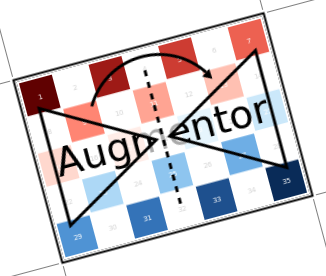It is also possible to pass some abstract vector to the constructor, in which case Augmentor will randomly sample one of its elements every time the operation is applied.

julia> Rotate(-10:10)
Rotate by θ ∈ -10:10 degree

julia> Rotate([-3,-1,0,1,3])
Rotate by θ ∈ [-3, -1, 0, 1, 3] degree

Input Sampled outputs for Rotate(-10:10)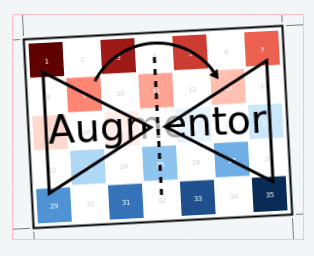##### Shearing¶
class ShearX

Shear the image horizontally for the given degree. This operation can only be described as an affine transformation and will in general cause other operations of the pipeline to use their affine formulation as well (if they have one).

It will always perform the transformation around the center of the image. This can be particularly useful when combining the operation with CropNative.

julia> ShearX(10)
ShearX 10 degree

Input Output for ShearX(10)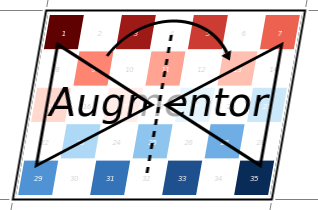It is also possible to pass some abstract vector to the constructor, in which case Augmentor will randomly sample one of its elements every time the operation is applied.

julia> ShearX(-10:10)
ShearX by ϕ ∈ -10:10 degree

julia> ShearX([-3,-1,0,1,3])
ShearX by ϕ ∈ [-3,-1,0,1,3] degree

Input Sampled outputs for ShearX(-10:10)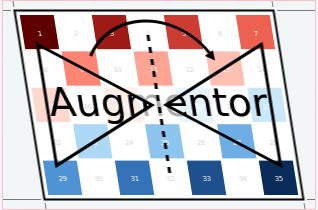class ShearY

Shear the image vertically for the given degree. This operation can only be described as an affine transformation and will in general cause other operations of the pipeline to use their affine formulation as well (if they have one).

It will always perform the transformation around the center of the image. This can be particularly useful when combining the operation with CropNative.

julia> ShearY(10)
ShearY 10 degree

Input Output for ShearY(10)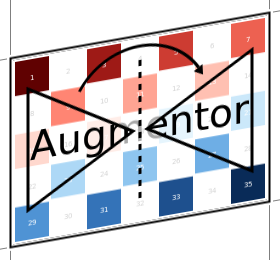It is also possible to pass some abstract vector to the constructor, in which case Augmentor will randomly sample one of its elements every time the operation is applied.

julia> ShearY(-10:10)
ShearY by ψ ∈ -10:10 degree

julia> ShearY([-3,-1,0,1,3])
ShearY by ψ ∈ [-3, -1, 0, 1, 3] degree

Input Sampled outputs for ShearY(-10:10)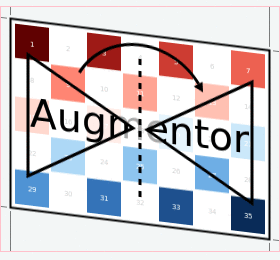##### Scaling¶
class Scale

Multiplies the image height and image width by individually specified constant factors. This means that the size of the output image depends on the size of the input image.

julia> Scale(0.9,0.5)
Scale by 0.9×0.5

Input Output for Scale(0.9,0.5)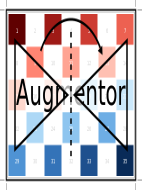In the case that only a single scale factor is specified, the operation will assume that the intention is to scale all dimensions uniformly by that factor.

julia> Scale(1.2)
Scale by 1.2×1.2

Input Output for Scale(1.2)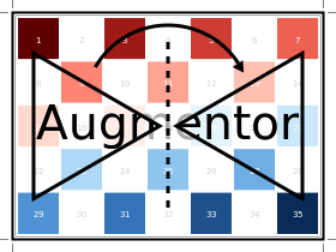It is also possible to pass some abstract vector(s) to the constructor, in which case Augmentor will randomly sample one of its elements every time the operation is applied.

julia> Scale([1.1, 1.2], [0.8, 0.9])
Scale by I ∈ {1.1×0.8, 1.2×0.9}

julia> Scale([1.1, 1.2])
Scale by I ∈ {1.1×1.1, 1.2×1.2}

julia> Scale(0.9:0.05:1.2)
Scale by I ∈ {0.9×0.9, 0.95×0.95, 1.0×1.0, 1.05×1.05, 1.1×1.1, 1.15×1.15, 1.2×1.2}

Input Sampled outputs for Scale(0.9:0.05:1.3)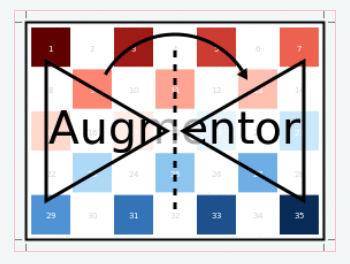class Zoom

Multiplies the image height and image width by individually specified constant factors. In contrast to Scale, the size of the input image will be preserved. This is useful to implement a strategy known as “scale jitter”.

julia> Zoom(1.2)
Zoom by 1.2×1.2

Input Output for Zoom(1.2)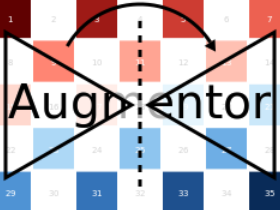It is also possible to pass some abstract vector to the constructor, in which case Augmentor will randomly sample one of its elements every time the operation is applied.

julia> Zoom([1.1, 1.2], [0.8, 0.9])
Zoom by I ∈ {1.1×0.8, 1.2×0.9}

julia> Zoom([1.1, 1.2])
Zoom by I ∈ {1.1×1.1, 1.2×1.2}

julia> Zoom(0.9:0.05:1.2)
Zoom by I ∈ {0.9×0.9, 0.95×0.95, 1.0×1.0, 1.05×1.05, 1.1×1.1, 1.15×1.15, 1.2×1.2}

Input Sampled outputs for Zoom(0.9:0.05:1.3)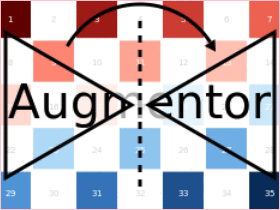#### Distorting¶

class ElasticDistortion
Input Sampled outputs for ElasticDistortion(15,15,0.1)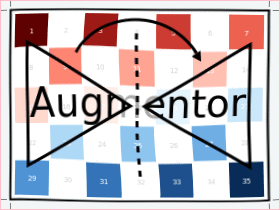Input Sampled outputs for ElasticDistortion(10,10,0.2,4,3,true)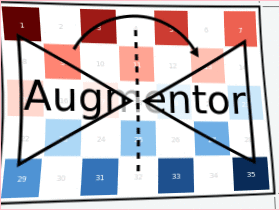#### Resizing and Subsetting¶

The process of cropping is useful to discard parts of the input image. To provide this functionality lazily, applying a crop introduces a layer of representation called a “view” or SubArray. This is different yet compatible with how affine operations or other special purpose implementations work. This means that chaining a crop with some affine operation is perfectly fine if done sequentially. However, it is generally not advised to combine affine operations with crop operations within an Either block. Doing that would force the Either() to trigger the eager computation of its branches in order to preserve type-stability.

##### Cropping¶
class Crop

Crops out the area of the specified pixel dimensions starting at a specified position, which in turn denotes the top-left corner of the crop. A position of x = 1, and y = 1 would mean that the crop is located in the top-left corner of the given image

julia> Crop(1:10, 5:20)
Crop region 1:10×5:20

julia> Crop(5, 1, 20, 10)
Crop region 1:10×5:24

Input Output for Crop(70:140,25:155)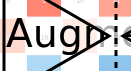class CropNative

Crops out the area of the specified pixel dimensions starting at a specified position. In contrast to Crop, the the position (1,1) is not located at the top left of the current image, but instead depends on the previous transformations. This is useful for combining transformations such as Rotation or ShearX with a crop around the center area.

julia> CropNative(1:10, 5:20)
Crop native region 1:10×5:20

Output for (Rotate(45), Crop(1:210,1:280)) Output for (Rotate(45), CropNative(1:210,1:280))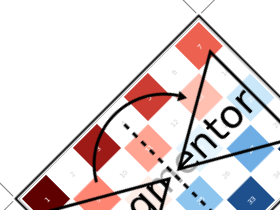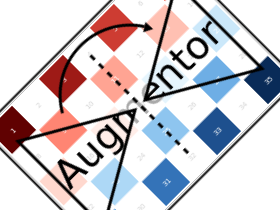class CropSize

Crops out the area of the specified pixel dimensions around the center of the given image.

julia> CropSize(45,250)
Crop a 45×250 window around the center

Input Output for CropSize(45,225)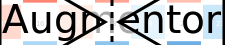class CropRatio

Crops out the biggest area around the center of the given image such that the output image satisfies the specified aspect ratio (i.e. width divided by height).

For example the operation CropRatio(1) would denote a crop for the biggest square around the center of the image, while CropRatio(16/9) would result in a rectangle with 16:9 aspect ratio.

julia> CropRatio(1)
Crop to 1:1 aspect ratio

julia> CropRatio(2.5)
Crop to 5:2 aspect ratio

Input Output for CropRatio(1)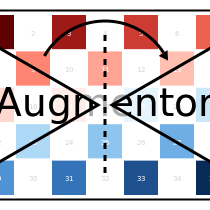class RCropRatio

Crops out the biggest possible area at some random position of the given image, such that the output image satisfies the specified aspect ratio (i.e. width divided by height).

For example the operation RCropRatio(1) would denote a crop for the biggest possible square. If there is more than one such square, then one will be selected at random.

julia> RCropRatio(1)
Crop random window with 1:1 aspect ratio

julia> CropRatio(2.5)
Crop random window with 5:2 aspect ratio

Input Sampled outputs for RCropRatio(1)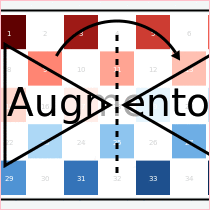##### Resizing¶
class Resize

Transforms the image into a fixed specified pixel size. This operation does not take any measures to preserve aspect ratio of the source image. Instead, the original image will simply be resized to the given dimensions. This is useful when one needs a set of images to all be of the exact same size.

julia> Resize(30,40)
Resize to 30×40

Input Output for Resize(100,150)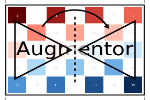#### Conversion¶

class ConvertEltype

Convert the element type of the given array/image into the given eltype. This operation is especially useful for converting color images to grayscale (or the other way around). That said the operation is not specific to color types and can also be used for numeric arrays (e.g. with separated channels).

Note that this is an element-wise convert function. Thus it can not be used to combine or separate color channels. Use SplitChannels or CombineChannels for those purposes.

julia> op = ConvertEltype(Gray)
Convert eltype to Gray

julia> img = testpattern()
300×400 Array{RGBA{N0f8},2}:
[...]

julia> augment(img, op)
300×400 Array{Gray{N0f8},2}:
[...]

Input Output for ConvertEltype(GrayA)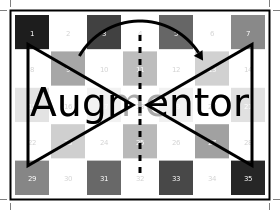#### Information Layout¶

It is not uncommon that machine learning frameworks require the data in a specific form and layout. For example many deep learning frameworks expect the colorchannel of the images to be encoded in the third dimension of a 4-dimensional array.

Augmentor allows to convert from (and to) these different layouts using special operations that are mainly useful in the beginning or end of a augmentation pipeline.

##### Color Channels¶
class SplitChannels

Separate the color channels of the given image into a dedicated array dimension. This will effectively create a new array dimension for the colors as the first dimension. In the case of greyscale images a singleton dimension will be created

This operation is mainly useful at the end of a pipeline in combination with PermuteDims in order to prepare the image for the training algorithm, which often requires the color channels to be separate.

julia> op = SplitChannels()
Split colorant into its color channels

julia> img = testpattern()
300×400 Array{RGBA{N0f8},2}:
[...]

julia> augment(img, op))
4×300×400 Array{N0f8,3}:
[...]

class CombineChannels

Combines the first dimension of a given array into a colorant of the specified type colortype. A separate color channel is also expected for Gray images.

The shape of the input image has to be appropriate for the given colortype, which also means that the separated color channel has to be the first dimension of the array. Use PermuteDims and/or Reshape if that is not the case.

This operation is mainly useful at the beginning of the pipline, if the colorchannels of the input images are separated.

julia> op = CombineChannels(RGB)
Combine color channels into colorant RGB{Any}

julia> A = rand(3, 10, 10) # random 10x10 RGB image
3×10×10 Array{Float64,3}:
[...]

julia> augment(A, op)
10×10 Array{RGB{Float64},2}:
[...]

##### Array Shape¶
class PermuteDims

Permute the dimensions of the given array with the predefined permutation perm. This operation is particularly useful if the order of the dimensions needs to be different than the default “julian” layout.

More concretely, Augmentor expects the given images to be in vertical-major layout for which the colors are encoded in the element type itself. Many deep learning frameworks however require their input in a different order. For example it is not untypical that the color channels are expected to be encoded in the third dimension.

julia> op = PermuteDims(3,2,1)
Permute dimension order to (3,2,1)

julia> img = testpattern()
300×400 Array{RGBA{N0f8},2}:
[...]

julia> augment(img, PermuteDims(2,1))
400×300 Array{RGBA{N0f8},2}:
[...]

class Reshape

Reinterpret the shape of the given array of numbers or colorants. This is useful for example to create singleton dimensions that deep learning frameworks may need for colorless images, or for converting an image to a feature vector and vice versa.

Note that this operation has nothing to do with image resizing, but instead is strictly concerned with changing the shape of the array.

julia> Reshape(10,15)
Reshape array to 10×15

julia> op = Reshape(25)
Reshape array to 25-element vector

julia> A = rand(5,5)
5×5 Array{Float64,2}:
[...]

julia> augment(A, op)
25-element Array{Float64,1}:
[...]


#### Utility Operations¶

Aside from “true” operations that specify some kind of transformation, there are also a couple of special utility operations used for functionality such as stochastic branching.

##### Buffering¶
class CacheImage

Write the current state of the image into the working memory. Optionally a user has the option to specify a preallocated buffer to write the image into.

Even without a preallocated buffer it can be beneficial to cache the image in some situations. For example when chaining a number of affine transformations after an elastic distortion, because performing that lazily requires nested interpolation.

julia> CacheImage()
Cache into temporary buffer

julia> CacheImage(rand(5,5))
Cache into preallocated 5×5 Array{Float64,2}

##### Identity Function¶
class NoOp

Pass the image along unchanged. Usually used in combination with Either to denote a “branch” that does not perform any computation.

julia> NoOp()
No operation

##### Stochastic Branches¶
class Either

Allows for choosing between different operations at random when applied. This is particularly useful if one for example wants to first either rotate the image 90 degree clockwise or anticlockwise (but never both) and then apply some other operation(s) afterwards.

When compiling a pipeline, Either will analyze the provided operations in order to identify the most preferred way to apply the individual operation when sampled, that is supported by all given operations. This way the output of applying Either will be inferable and the whole pipeline will remain type-stable, even though randomness is involved.

By default each specified image operation has the same probability of occurrence. This default behaviour can be overwritten by specifying the chance manually.

julia> FlipX() * FlipY()
Augmentor.Either (1 out of 2 operation(s)):
- 50% chance to: Flip the X axis
- 50% chance to: Flip the Y axis

julia> Either(FlipX(), FlipY())
Augmentor.Either (1 out of 2 operation(s)):
- 50% chance to: Flip the X axis
- 50% chance to: Flip the Y axis

julia> Either((FlipX(), FlipY(), NoOp()), (1,1,2))
Augmentor.Either (1 out of 3 operation(s)):
- 25% chance to: Flip the X axis
- 25% chance to: Flip the Y axis
- 50% chance to: No operation

julia> Either(1=>FlipX(), 1=>FlipY(), 2=>NoOp())
Augmentor.Either (1 out of 3 operation(s)):
- 25% chance to: Flip the X axis
- 25% chance to: Flip the Y axis
- 50% chance to: No operation

julia> (1=>FlipX()) * (1=>FlipY()) * (2=>NoOp())
Augmentor.Either (1 out of 3 operation(s)):
- 25% chance to: Flip the X axis
- 25% chance to: Flip the Y axis
- 50% chance to: No operation